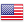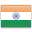Grade "A+" Accredited by NAAC with a CGPA of 3.46
Grade "A+" Accredited by NAAC with a CGPA of 3.46

# Data Structures

Course ID
BHCS 05
Level
Program
B.Sc. CS (Hons.)
Semester
Third
Credits
6.0
Paper Type
Core Course
Method
Lecture & Practical

## Unique Paper Code: Update Awaited

This course aims at developing the ability to use basic data structures like array, stacks, queues, lists, trees and hash tables to solve problems. C++ is chosen as the language to understand implementation of these data structures.

## Learning Outcomes:

At the end of the course, students should be able to:

• Implement and empirically analyse linear and non-linear data structures like Arrays, Stacks, Queues, Lists, Trees, Heaps and Hash tables as abstract data structures. (RBT L2/3)
• Write a program, choosing a data structure, best suited for the application at hand. (RBT L3/4)
• Re-write a given program that uses one data structure, using a more appropriate/efficient data structure (RBT L4)
• Write programs using recursion for simple problems. Explain the advantages and disadvantages of recursion.(RBT L2/L3)
• Identify Ethical Dilemmas.

## Course Contents

Unit 1
Unit 2
Unit 3
Unit 4
Unit 5
Unit 6

Unit 1

Arrays: single and multi-dimensional arrays, analysis of insert, delete and search operations in arrays (both linear search and binary search), implementing sparse matrices, applications of arrays to sorting: selection sort, insertion sort, bubble sort, comparison of sorting techniques via empirical studies. Introduction to Vectors.

Unit 2

Linked Lists: Singly- linked, doubly-linked and circular lists, analysis of insert, delete and search operations in all the three types, implementing sparse matrices. Introduction to Sequences.

Unit 3

Queues: Array and linked representation of queue, de-queue, comparison of the operations on queues in the two representations. Applications of queues.

Unit 4

Stacks: Array and linked representation of stacks, comparison of the operations on stacks in the two representations, implementing multiple stacks in an array; applications of stacks: prefix, infix and postfix expressions, utility and conversion of these expressions from one to another; applications of stacks to recursion: developing recursive solutions to simple problems, advantages and limitations of recursion.

Unit 5

Trees and Heaps: Introduction to tree as a data structure; binary trees, binary search trees, analysis of insert, delete, search operations, recursive and iterative traversals on binary search trees. Height-balanced trees (AVL), B trees, analysis of insert, delete, search operations on AVL and B trees.
Introduction to heap as a data structure. analysis of insert, extract-min/max and delete-min/max operations, applications to priority queues.

Unit 6

Hash Tables: Introduction to hashing, hash tables and hashing functions -insertion, resolving collision by open addressing, deletion, searching and their analysis, properties of a good hash function.

### Practicals

#### Lab List 1

1. Write a program to search an element from a list. Give user the option to perform Linear or Binary search. Use Template functions.
2. WAP using templates to sort a list of elements. Give user the option to perform sorting using Insertion sort, Bubble sort or Selection sort.
3. Implement Linked List using templates. Include functions for insertion, deletion and search of a number, reverse the list and concatenate two linked lists (include a function and also overload operator +).
4. Implement Doubly Linked List using templates. Include functions for insertion, deletion and search of a number, reverse the list.
5. Implement Circular Linked List using templates. Include functions for insertion, deletion and search of a number, reverse the list.
6. Perform Stack operations using Linked List implementation.
7. Perform Queues operations using Circular Array implementation. Use Templates.
8. Perform Queues operations using Circular Array implementation. Use Templates.
9. Create and perform different operations on Double-ended Queues using Linked List implementation.
10. WAP to scan a polynomial using linked list and add two polynomial.

#### Lab List 2

1. WAP to calculate factorial and to compute the factors of a given no. (i)using recursion, (ii) using iteration
2. (ii) WAP to display fibonacci series (i)using recursion, (ii) using iteration
3. Create a class Student containing fields for Roll No., Name, Class, Year and Total Marks. Write a program to store 5 objects of Student class in a file. Retrieve these records from file and display them.
4. WAP to calculate GCD of 2 number (i) with recursion (ii) without recursion
5. WAP to create a Binary Search Tree and include following operations in tree: (a) Insertion (Recursive and Iterative Implementation) (b) Deletion by copying (c) Deletion by Merging (d) Search a no. in BST (e) Display its preorder, postorder and inorder traversals Recursively (f) Display its preorder, postorder and inorder traversals Iteratively (g) Display its level-by-level traversals (h) Count the non-leaf nodes and leaf nodes (i) Display height of tree (j) Create a mirror image of tree (k) Check whether two BSTs are equal or not
6. WAP to convert the Sparse Matrix into non-zero form and vice-versa.
7. WAP to reverse the order of the elements in the stack using additional stack.
8. WAP to reverse the order of the elements in the stack using additional Queue.
9. WAP to implement Diagonal Matrix using one-dimensional array.
10. WAP to implement Lower Triangular Matrix using one-dimensional array.
11. WAP to implement Upper Triangular Matrix using one-dimensional array.

#### Lab List 3

1. WAP to implement Symmetric Matrix using one-dimensional array.
2. WAP to create a Threaded Binary Tree as per inorder traversal, and implement operations like finding the successor / predecessor of an element, insert an element, inorder traversal.
3. WAP to implement various operations on AVL Tree.
4. WAP to implement heap operations.

#### Text Books

Drozdek, A., (2012), Data Structures and algorithm in C++. 3rd edition. Cengage Learning.
Goodrich, M., Tamassia, R., & Mount, D., (2011). Data Structures and Algorithms Analysis in C++. 2nd edition. Wiley.

Foruzan, B.A. (2012) Computer Science: A Structured Approach Using C++, Cengage Learning
Lafore, R. (2008). Object Oriented Programming in C++. 4th edition. SAMS Publishing.
Sahni, S. (2011). Data Structures, Algorithms and applications in C++. 2ndEdition, Universities Press
Tenenbaum, A. M., Augenstein, M. J., & Langsam Y., (2009), Data Structures Using C and C++. 2nd edition. PHI.

#### Teaching Learning Process

Use of ICT tools in conjunction with traditional class room teaching methods
Interactive sessions
Class discussions

#### Assessment Methods

Written tests, assignments, quizzes, presentations as announced by the instructor in the class

#### Keywords

Arrays and linked lists, stacks, queues, tree, heap, hashing, recursion.

Disclaimer: Details on this page are subject to change as per University of Delhi guidelines. For latest update in this regard please refer to the University of Delhi website here.Englishहिन्दी